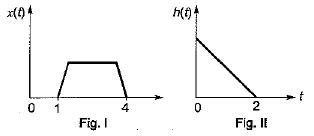Courses

# Introduction To Systems

## 10 Questions MCQ Test Mock Tests Electronics & Communication Engineering GATE 2020 | Introduction To Systems

Description
This mock test of Introduction To Systems for GATE helps you for every GATE entrance exam. This contains 10 Multiple Choice Questions for GATE Introduction To Systems (mcq) to study with solutions a complete question bank. The solved questions answers in this Introduction To Systems quiz give you a good mix of easy questions and tough questions. GATE students definitely take this Introduction To Systems exercise for a better result in the exam. You can find other Introduction To Systems extra questions, long questions & short questions for GATE on EduRev as well by searching above.
QUESTION: 1

Solution:
QUESTION: 2

Solution:
QUESTION: 3

### The unit impulse response of a linear time invariant system is the unit step function u(t) for t > 0, the response of the system to an excitation e-at u(t), a > 0 will be

Solution:
QUESTION: 4

A system with an input x(t) and output y(t) is described by the relation: y(t) = tx(t). This system is

Solution:
QUESTION: 5

If a function f(t) u(t) is shifted to the right side by t0, then the function can be expressed as

Solution:
QUESTION: 6

The impulse response of a causal, linear, time- invariant, continuous time system is h(t). The output y(t) of the same system to an input x(t). Where x(t) = 0 for t < -2 is

Solution:
QUESTION: 7

The unit step response of a system is given by (1 - eαt) u(t), the impulse response is given by

Solution:
QUESTION: 8

Figure I and Figure II, shows the input x(t) to a linear time invariant system and the impulse response h(t) of the systemthe output of the system is zero everywhere except for the time interval.

Solution:
QUESTION: 9

A signal f(t) = cos8πt + 0.5cos4πt is instantaneously sampled. The maximum allowable value of sampling interval Ts in sec is

Solution:
QUESTION: 10

The impulse response. h[n] of a linear time invariant system is given by h[n] = u[n + 3] + u[n - 3] - 2u[n - 7], the above system is

Solution: